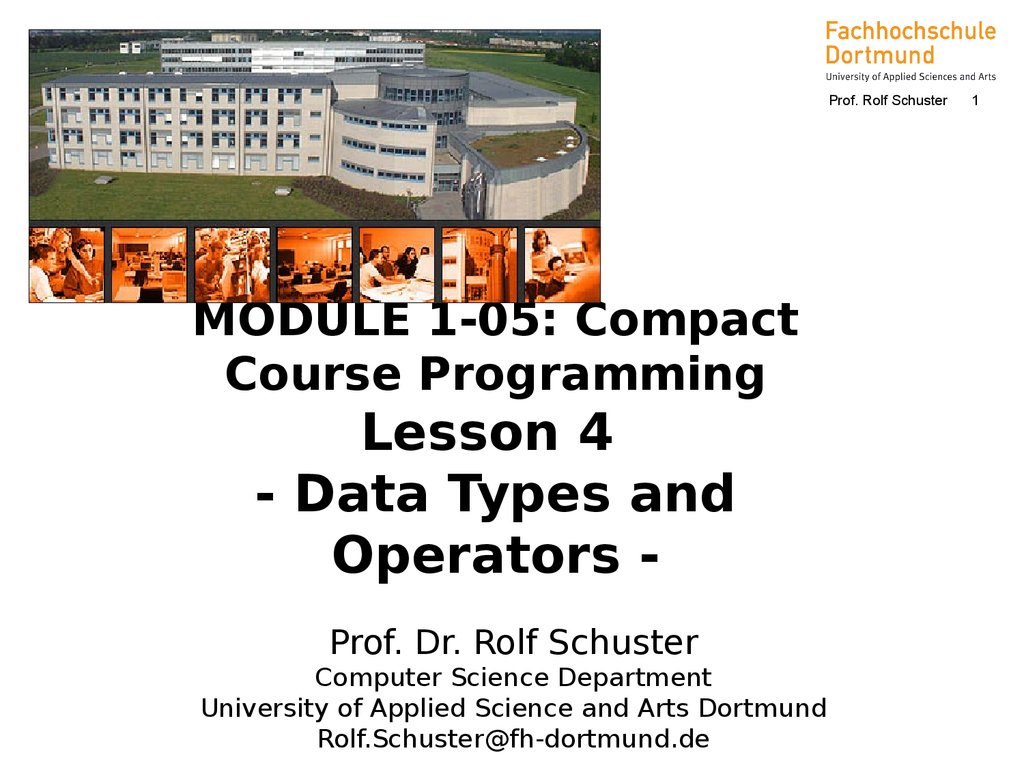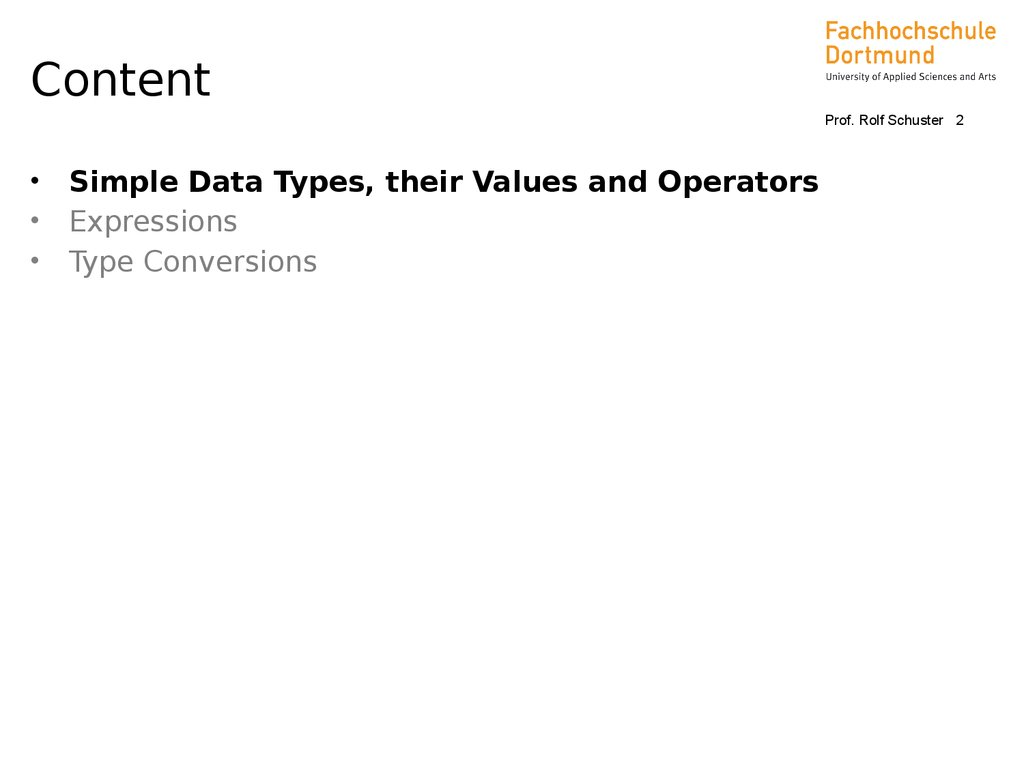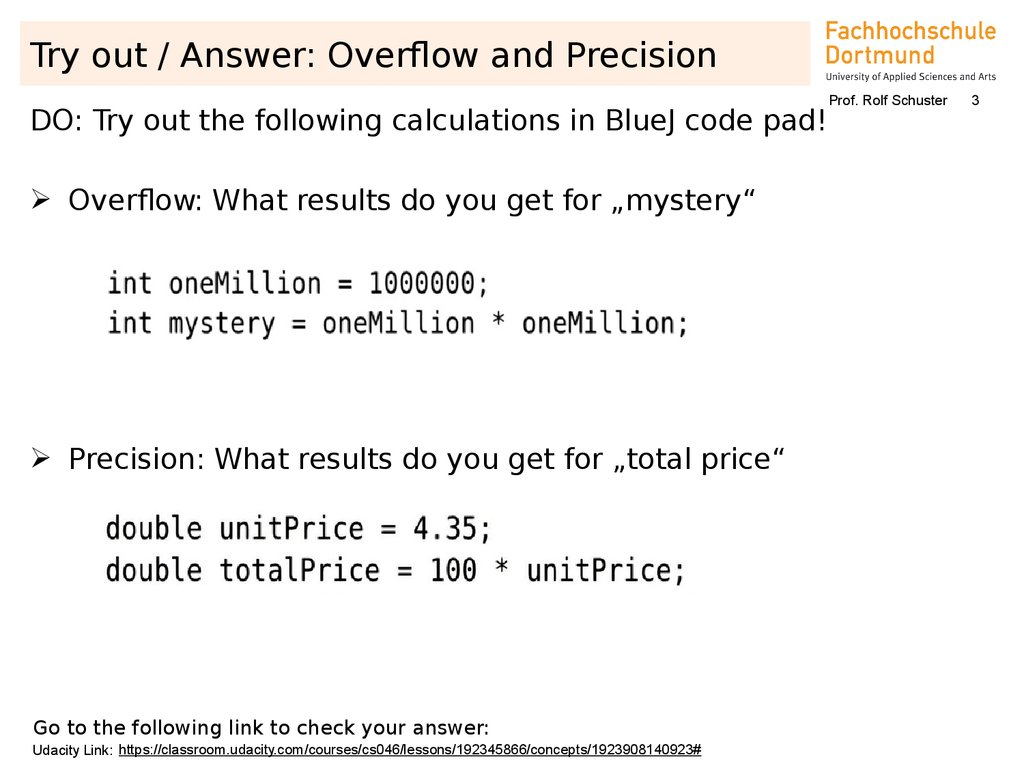# Data Types and Operators (Java, Lecture 04)

## 1.

Prof. Rolf Schuster
MODULE 1-05: Compact
Course Programming
Lesson 4
- Data Types and
Operators Prof. Dr. Rolf Schuster
Computer Science Department
University of Applied Science and Arts Dortmund
[email protected]
1

## 2. Content

Prof. Rolf Schuster 2
Simple Data Types, their Values and Operators
Expressions
Type Conversions

## 3. Try out / Answer: Overflow and Precision

DO: Try out the following calculations in BlueJ code pad!
Overflow: What results do you get for „mystery“
Precision: What results do you get for „total price“
Prof. Rolf Schuster
3

## 4. Simple Data Types, their Values and Operators Simple Data Types in Java

Prof. Rolf Schuster 4
Type
Name
Content
Value Range
DefaultValue
Size
boolean
Locic Value
true, false
false
1 Bytes
char
UnicodeCharacter
\u0000 … \uffff
(0
… 65535)
\u0000
2 Bytes
byte
Whole Number
with +/- Sign
-128 … 127
0
1 Bytes
short
Whole Number
with +/- Sign
-32768 … 32767
0
2 Bytes
int
Whole Number
with +/- Sign
-2147483648 … 2147483647
0
4 Bytes
long
Whole Number
with +/- Sign
-9223372036854775808 …
0
8 Bytes
9223372036854775807
float
Floating Point
Number
+/- 3.4028235 1038
appr. 7 significant decimal places
0.0f
4 Bytes
double
Floating Point
Number
+/-1.7976931348623157 10308
appr. 7 significant decimal places
0.0d
8 Bytes

## 5. Simple Data Types, their Values and Operators Simple Data Types in Java

Prof. Rolf Schuster 5
In difference to some other programming languages, all simple
data types in Java have an agreed fixed size in memory
For every simple data type a default value is defined, which is of
importance with the initialisation of object and class variables
(note: local variables are not automatically initialised with the
default value)

## 6. Simple Data Types, their Values and Operators Operators

Prof. Rolf Schuster 6
Are special symbols that are used to link operands to determine a
new value
According to their number of operands we can distinguish three
types of operators
– Single digit (monadic oder unary) operators
example: the negative sign – Two digit (dyadic oder binary) operators
– Three digit (triadic oder ternary) operatores
example: conditional operator ? :

## 7. Simple Data Types, their Values and Operators Logic Values (boolean)

Prof. Rolf Schuster 7
Unary Operator (Operator Operand)
Operator Description
!
logische Negation
Binary Operator (Operand1 Operator Operand2)
Operator Description
Example
== Equality
false == true results in false
!= Inequality
false != true results in true
& logic AND
| logic OR
^ logic XOR

## 8. Simple Data Types, their Values and Operators Logic Value (boolean)

Prof. Rolf Schuster 8
Notation
Area
NEGATION AND
OR
Exklusiv-OR
Mathematics / a
Logic
a b
a b
a b
Java
a&b
a|b
a^b
!a
Properties of the Operators (truth table)
a
!a
a
b
a&b
a|b
a^b
false
true
false
false
false
false
false
true
false
false
true
false
true
true
true
false
false
true
true
true
true
true
true
false

## 9. Simple Data Types, their Values and Operators Truth Table (boolean)

Prof. Rolf Schuster 9
Boolean Expressions with & and | evaluate both terms completely
In practise complete evaluation is often not required
– & - operation: is one expression false, then the overall result is
false
– | - operation: is one expression true, then the overall result is
true
The operators && and || ensure a shortened evaluation
n>3 is not evaluated!
Example
int m = 3;
int n = 5;
boolean b = (m < 5) || (n > 3);
The shortened evaluation is important to reduce the
computation time for example with large arrays

## 10. Try out / Answer: use boolean operators!

Prof. Rolf Schuster
What is the value of the following expressions?
1.
2.
3.
4.
(true & true) | false
!!true
true & !true
(true || false) && true
10

## 11. Simple Data Types, their Values and Operators Binary Operators (char, byte, short, int, long)

Operator Description
Example
5+6
yields 11
- Subtraction
9-3
yields 6
* Multiplication
10 * 15
yields 150
/ Whole Number Division 13 / 3
yields 4
% Modulo (remainder of
20 % 7
yields 6
a whole number division)
< smaller
3<5
yields true
<= smaller equal
3 <= 3
yields true
> bigger
2 > 10
yields false
>= bigger equal
5 >= 6
yields false
== equal
3 == 3
yields true
!= not equal
5 != 5 yields false
Prof. Rolf Schuster 11

## 12. Try out / Answer: use boolean operators!

Prof. Rolf Schuster
What is the value of the following expressions?
1.
2.
3.
4.
7
7
5
5
/
%
/
%
5
5
7
7
12

## 13. Simple Data Types, their Values and Operators Unary Operators (char, byte, short, int, long)

Prof. Rolf Schuster 13
Operator Description
Example
Unary Negation
-i
++
Increment
++i is the same as i = i+1
-Decrement
--i is the same as i = i-1
Note the difference: Pre-increment vs. Post-increment
a = ++b;
// is the same as:
// b = b+1; a = b;
a = b++;
// is the same as:
// a = b; b = b+1;

## 14. Simple Data Types, their Values and Operators Bitwise - Operators (char, byte, short, int, long)

Prof. Rolf Schuster 14
Numbers are viewed as a set of consecutive bits, which may be
manipulated
Unary Operator (Operator Operand)
Operator
Description
~
Complement (bitwise negation)
Binary Operators (Operand1 Operator Operand2)
Operator
Description
&
bitwise AND
|
bitwise OR
^
bitwise XOR
The operators >>, >>> and << are used to shift the bits to the right or
the left

## 15. Simple Data Types, their Values and Operators Bitwise - Operators (char, byte, short, int, long)

Prof. Rolf Schuster 15
Example for shift operator
– Left-Shift-Operator <<
lost bits
int a;
a = 10;
00000000 00000000 00000000 00001010
a << 3; 00000000 00000000 00000000 01010000
filled with bits
int a;
lost bits
a = -10;
11111111 11111111 11111111 11110110
a << 3; 11111111 11111111 11111111 10110000
filled with bits
Equivalent to: whole-number multiplication with 23

## 16. Simple Data Types, their Values and Operators Floating-Point-Numbers (float, double)

Prof. Rolf Schuster 16
Unary Operators (analog to whole-number types)
++ -Binary Operators (analog to whole-number types)
+ - * / % (arithmetic operators)
<
<=
>
>=
==
!= (comparison operators)
Note:
– whole-number division: 45 / 20
Result: 2
– floating-point division:
45.0 / 20.0
Result: 2.25

## 17. Simple Data Types, their Values and Operators Floating-Point-Numbers (float, double)

Arithmetic Operators
– Both operands of type float
• Result type float
– In all other cases
• Result type double
Attention with equality checks
(x == y)
// possible rounding errors !
Prof. Rolf Schuster 17

## 18. Simple Data Types, their Values and Operators Composite Operators

E1 op= E2
is the same as
Prof. Rolf Schuster 18
E1 = E1 op (E2)
Example
counter = counter + 1;
// abbreviated: counter += 1;
counter = counter – 1;
// abbreviated: counter –= 1;
– analog: *=, /=, %=, &=, |=, ^=, <<=, >>=, >>>=

## 19. Content

Prof. Rolf Schuster 19
Simple Data Types, their Values and Operators
Expressions
Type Conversions

## 20. Expressions Expressions: Definition and Features

Prof. Rolf Schuster 20
Expression: Processing specification, that delivers a value after
execution
In the simplest case a variable or a constant
Through combination of operands, operations and round
brackets we get complex expressions
Examples
counter = counter + 1;

## 21. Expressions Brackets

Prof. Rolf Schuster 21
The evaluation of expressions in brackets always takes place first
– Just like the rules in mathematics
Expressions may be arbitrarily nested
Notation of the nested structure is done with round brackets
All expressions are provided in linear notation
Expression are provided in line format

## 22. Expressions Example

Prof. Rolf Schuster 22
Mathematic format
k t p
100 360
Line format
k * t * p / (100 * 360)
a f c d
a e b d
(a * f + c * d) / (a * e – b * d)
b
a
d
e
f
g
h
p
B0 1 n
100
a + b / (d + e / (f + g / h))
B0 * (1 – n * p / 100)

## 23. Expressions Operator Priority Rules

Prof. Rolf Schuster 23
Well-known from mathematics:
– „Point before Line“
• example 6 + 7 * 3 equals 27 and not 39
In Java:
– Linking of operators is governed by priorities:
• An operator with high priority links stronger than an
operator with a lower priority
• If the priority is the same than then the associativity of
the operators is evaluated
– op is is left associative: X op Y op Z equals (X op Y) op
Z
– op ist right associative: X op Y op Z equals X op (Y op
Z)
• Obviously, brackets do control the evaluation order
– example (6 + 7) * 3 ist 39

## 24. Expressions Priority and Associativity

Priority
Prof. Rolf Schuster 24
Operators
[]
Description
Associativity
Field(Array)index
L
()
Method call
L
.
Component access
L
++ --
Pre- oder post-increment or –decrement
R
+ -
Sign (unary)
R
~
Bitwise complement
R
!
Logic negation
R
(type)
Type conversion
R
new
Object generation
R
12
* / %
Multiplication, division, modulo
L
11
- +
L
10
<<
Left shift
L
>>
Right shift with sign
L
14
13

## 25. Expressions Priority and Associativity

Prof. Rolf Schuster 25
Priority
Operators
Description
Associativity
9
< <= > >=
Comparison: smaller, smaller or equal, bigger,
bigger or equal
L
instanceof
Type check of object
L
8
== !=
Equal, not equal
L
7
&
Logic-, bitwise AND
L
6
^
Logic-, bitwise Exclusiv-OR
L
5
|
Logic-, bitwise OR
L
4
&&
Logic AND
L
3
||
Logic OR
L
2
? :
Conditioned evaluation
L
1
=
Assignment
R
*= /= %=
Combined assignment
R
+= -= <<=
R
>>= >>>=
R
&= ^= |=
R

## 26. Try out / Answer: use priority and associativity!

Prof. Rolf Schuster
For the following expressions, set brackets such that they yield
the same result as the expressions without brackets
1. e = --c - d / a;
2. f = b <= a || c > 16;
3. h = a < 5 || b > 10 && d - c >= 0;
26

## 27. Expressions Mathematical Constants and Functions

Prof. Rolf Schuster 27
The class Math provides important mathematical constants and
functions (see online documentation)
Constants
– public static final double E (basis e of nat. logarithm)
– public static final double PI
( )
– Usage: Math.E and Math.PI
Methods (Selection)

public static double abs(double x)
|x|
public static double cos(double x)
cos(x)
public static double sin(double x)
sin(x)
public static double tan(double x)
tan(x)
public static double sqrt(double x)
√x
public static double exp(double x)
ex
public static double pow(double x, double y)
xy
Usage: for example result = Math.pow(a,b);

## 28. Definition of Constants

Prof. Rolf Schuster
Constants in Java
• Constants are defined and initialised like variables with the keyword
„final“
• their names are typically written in capital letters
• they can not be changed
Example:
Statement and definition of constants:
Statement rewritten with constants:
red = Math.min(red + ADDED_RED, MAX_RED)
28

## 29. Content

Prof. Rolf Schuster 29
Simple Data Types, their Values and Operators
Expressions
Type Conversions

## 30. Type Conversion Type Conflict

Prof. Rolf Schuster 30
Values can only be assigned to variables,
if their type is compatible with the type of the variable!
Example
int a;
float b = 10.5f;
a = b; // Error because of incompatible types

## 31. Type Conversion Automatic (implicit) type extension

Prof. Rolf Schuster 31
Rules
byte
short int
char
long
float
double
– An automatic type extension is happening in the direction of
the arrows
Example
double a, b;
float c;
a = b + c + 2.785f;

## 32. Type Conversion Type extension and selection of operators in expressions

Prof. Rolf Schuster 32
Each expression is evaluated step by step according to the
priorities and associativity of its operators
The operators choosen are the operators that fit to the type of
the operands
(example: whole number division OR division for floating point
numbers)
Are the types of operands different, than the „smaller“ operand
will receive an automatic type conversion
Are both operands of an operation, expressions themselves, then
the left operand is calculated before the right operand

## 33. Type Conversion Type Extension and Selection of Operators in Expressions

Prof. Rolf Schuster 33
• Example
double a;
int b, c;
a = 3.0 + 2.785f + b / c;
• Evaluation: Addition from left to right, point before line
3) Addition of double- und int-value: int-value extended to double and + for double
a = ((3.0 + 2.785f)
+
1) 3.0 double, 2.785f float => type extension
to double 2.785 and + for double-values
(b / c));
2) Both operands of type int
=> whole number division

## 34. Type Conversion Explicit Type Conversion: Type Casting

Prof. Rolf Schuster 34
Explicit type conversion happens when the desired type is
explicitly requested
Example
int a;
float b = 10.25f;
a = (int) b;
float-value 10.25f is converted in the int-value 10
a = (int)(b / 3.3f + 5.73f);
Note: Type casting takes place AFTER the calculation of the
entire term
b / 3.3f + 5.73f

## 35. Reading Input from the Console

Prof. Rolf Schuster
Example code to read an integer and a double from the keyboard:
Remember to import the utilisation class „Scanner“
import java.util.Scanner;
public class InputDemo
{
public static void main(String[] args)
{
Scanner in = new Scanner(System.in);
System.out.print("How old are you? ");
int age = in.nextInt();
Imports the
Scannerclass
integer from
the console
System.out.print("Next year, you will be ");
System.out.println(age + 1);
double weight = in.nextDouble();
System.out.print("I hope next year that'll be ");
System.out.print(weight * 0.95);
}
}
35
double from
the console

## 36. Formatted Output

Example code to print a number in a specific format:
public class FormatDemo
{
public static void main(String[] args)
{
int quantity = 100;
double unitPrice = 4.35;
double totalPrice = quantity * unitPrice;
System.out.print("Total: ");
System.out.printf("%8.2f\n", totalPrice);
double taxRate = 0.08;
double tax = totalPrice * taxRate;
System.out.print("Tax:
");
System.out.printf("%8.2f\n", tax);
}
}
Prof. Rolf Schuster
36
Printf-Formatting
with argument
„%8.2f\n“:
% - print something
8 - print total of 8
digits
.2 - with 2 digits after
the
decimal point
f - floating point
number
\n - print a new line

## 37. Try out / Answer: Overflow and Precision

DO: Fill in the empty fields!
Refer to the fact sheet for further details:
https://www.udacity.com/wiki/cs046/factsheets
Prof. Rolf Schuster
37

## 38. Homework Assignment 04 (2 Bonus Points) (Assignment submission date provided in „Ilias … Homework Assignments“

Homework Assignment 04
(2 Bonus Points)
(Assignment submission date provided in „Ilias … Homework
Assignments“
Write the program “Milage Printer” in
BlueJ….
Prof. Rolf Schuster
38
Sample runs for the final version:
Enter the number of gallons of gas in
…that asks the user to input the following the tank 5.1
Enter the fuel efficiency 35.0
values:
The number of gallons currently in the Distance: 178.5
tank
Cost:
11.29
The fuel efficiency in miles per gallon
and then prints how far the car can go on Or:
the gas
Enter the number of gallons of gas in
in the tank and the cost of driving 100
the tank 25
miles.
Enter the fuel efficiency -5
Print
thethe
distance
with
1 decimal
point
and No
can
Assume
cost per
gallon
is \$3.95.
Define
it as
a go
constant: final double
the
cost
with
2
decimals
COST_PER_GALLON = 3.95;
Use
System.out.print
and not is less than or equal to 0, print "No can go".
If value
entered for efficiency
System.out.println.